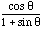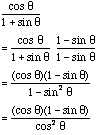Sender: Stu Date: Tue, 1 Jun 1999 21:15:17 -0700 Math 12 Student cos(theta) / 1+ sin(theta)=sec(theta)-tan(theta) I've being having trouble with this one on my correspondance course. Hi Stu, You didn't give any instructions but I expext that you want to show that the exprression is valid. Much of algebra and trigonometry is recognizing patterns. In the expressionThe "pattern" that I see is the 1+sin(theta) in the denominator I know that I can change it to 1-sin2(theta) by multiplying by 1-sin(theta), and that 1-sin2(theta) = cos2(theta). Thus my argument would proceed asYou should now be able to complete the problem. Cheers Harley Go to Math Central To return to the previous page use your browser's back button.